# How much tracing dye do I need to use?

Shop for Tracing Dyes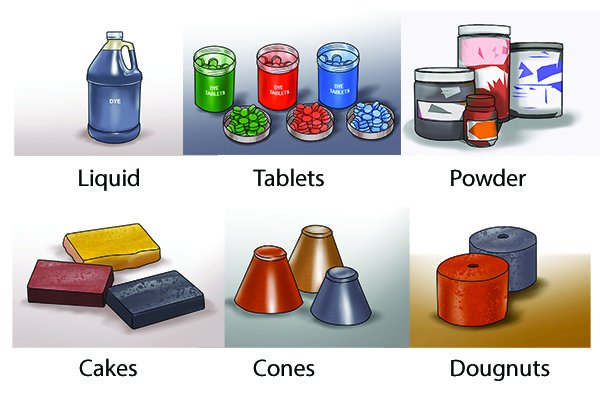The amount of dye you need varies depending on the clarity of the water with which you are working and according to the format of the dye – whether it’s powder, liquid, sachets, tablets, cakes, cones or doughnuts.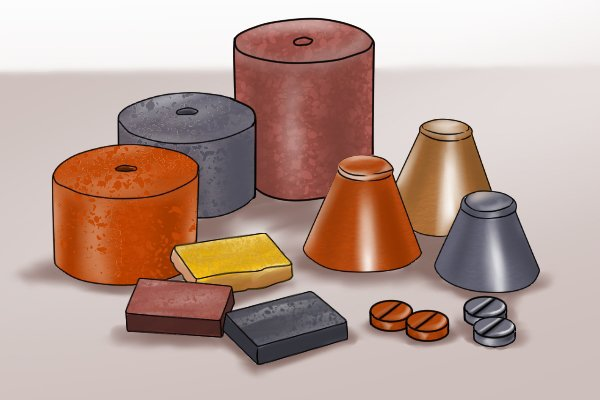There is also variance between dyes from the different manufacturers. For instance, one tracing dye maker claims its dyes are 40 per cent more concentrated than most others, so you need less of it for a given volume of water.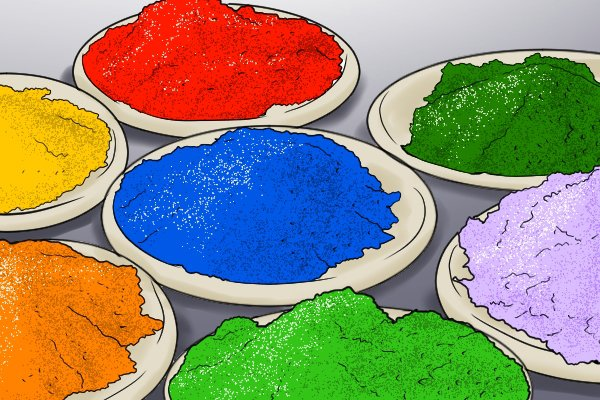The minimum traceable concentration of dyes in clear water is around 1ppm (parts per million), but the water is often discoloured, so much higher concentrations are needed for a clearly defined color change.

## Examples of dilution rates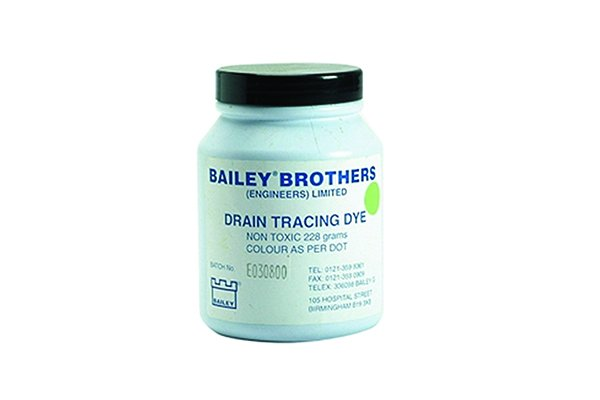200g (8oz) of powder dye from one manufacturer should give a strong visual trace in 15,000 litres (3,300 gallons) of water.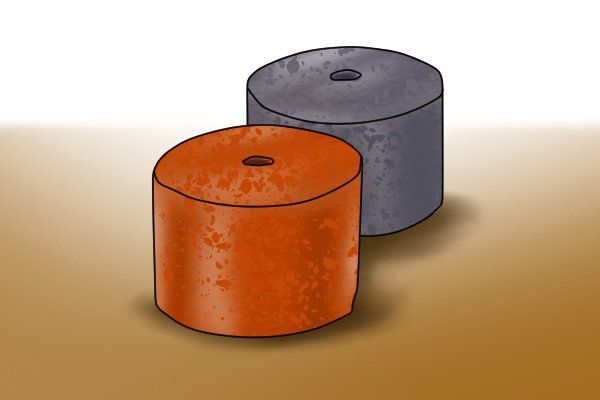As another example, doughnuts – the largest of the pressed powder varieties – from a particular manufacturer weigh 900g (32oz)  and will create a strong visual in around 27,300 litres (6,000 gallons) of water.

## How to know how much water you’re working with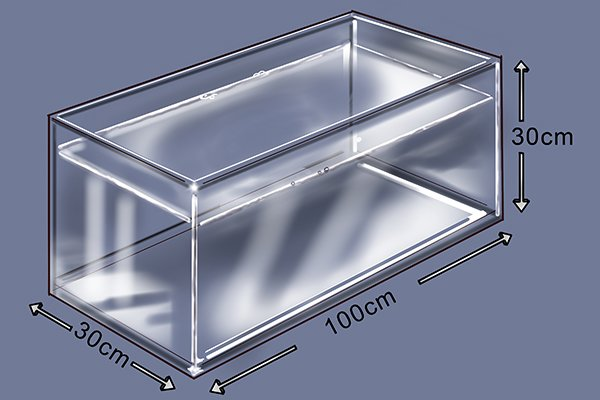There are ways to work out a volume of water such as Average depth x Average width x Average length = Total volume of water.  For example, to work out your total volume of water in centimetres and litres.

• Average depth = 30cm
• Average width = 30cm
• Average length = 100cm

30 x 30 x 100 = 90,000 cubic centimetres of water. To covert to litres you would divide this by 1000 giving you a total volume of water of 90 litres.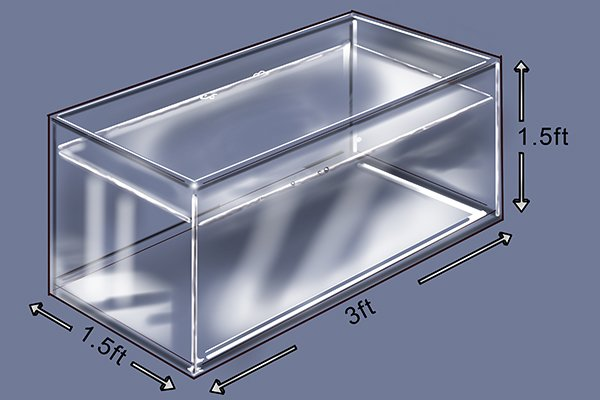If you want to work out your total volume of water in feet and gallons:

• Average depth = 1.5 feet
• Average width = 1.5 feet
• Average length = 3 feet

1.5 x 1.5 x 3 = 6.75 cubic feet of water. There are 6 gallons per cubic foot of water so you need to multiply this number by 6 giving you a total volume of water of 40.5 gallons.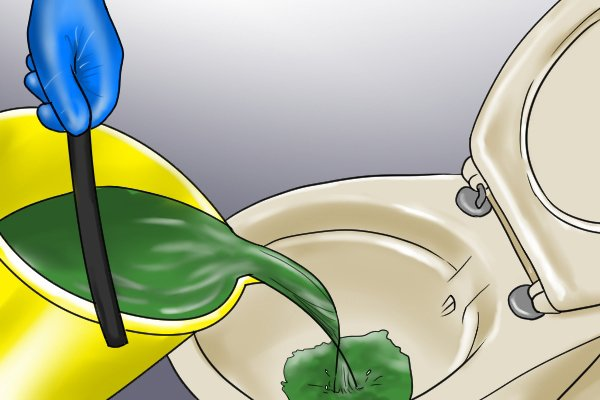Although these equations are useful to know, it is often easier to use trial and error, which is a simple way of saying pour some dye down the hole and if it is still visible when it comes out the other end then you used the right amount of dye.